# Positive solution for a class of singular semipositone fractional differential equations with integral boundary conditions

## Abstract

In this article, by employing a fixed point theorem in cones, we investigate the existence of a positive solution for a class of singular semipositone fractional differential equations with integral boundary conditions. We also obtain some relations between the solution and Green’s function.

MSC: 26A33, 34B15, 34B16, 34G20.

## 1 Introduction

In this article, we consider the existence of a positive solution for the following singular semipositone fractional differential equations:

$\left\{\begin{array}{c}{D}_{0+}^{\alpha }u\left(t\right)+f\left(t,u\left(t\right)\right)=0,\phantom{\rule{1em}{0ex}}0
(1)

where $f\in C\left[\left(0,1\right)×\left[0,+\mathrm{\infty }\right),\left(-\mathrm{\infty },+\mathrm{\infty }\right)\right]$, $3<\alpha \le 4$, $0<\eta \le 1$, $0\le \frac{\lambda {\eta }^{\alpha }}{\alpha }<1$, ${D}_{0+}^{\alpha }$ is the standard Riemann-Liouville derivative, $f\left(t,u\right)$ may be singular at $t=0$ and/or $t=1$. Since the nonlinearity $f\left(t,x\right)$ may change sign, the problem studied in this paper is called the semipositone problem in the literature which arises naturally in chemical reactor theory. Up to now, much attention has been attached to the existence of positive solutions for semipositone differential equations and the system of differential equations; see  and references therein to name a few.

Boundary value problems with integral boundary conditions for ordinary differential equations arise in different fields of applied mathematics and physics such as heat conduction, chemical engineering, underground water flow, thermo-elasticity, and plasma physics. Moreover, boundary value problems with integral conditions constitute a very interesting and important class of problems. They include two-point, three-point, multi-point, and nonlocal boundary value problems as special cases, which have received much attention from many authors. For boundary value problems with integral boundary conditions and comments on their importance, we refer the reader to the papers by Gallardo , Karakostas and Tsamatos , Lomtatidze and Malaguti , and the references therein.

On the other hand, fractional differential equations have been of great interest for many researchers recently. This is caused both by the intensive development of the theory of fractional calculus itself and by the applications of such constructions in various fields of science and engineering such as control, porous media, electromagnetic, and other fields. For an extensive collection of such results, we refer the readers to the monographs by Samko et al., Podlubny  and Kilbas et al.. For the case where α is an integer, a lot of work has been done dealing with local and nonlocal boundary value problems. For example, in  Webb studied the n th-order nonlocal BVP

$\left\{\begin{array}{c}{u}^{\left(n\right)}\left(t\right)+a\left(t\right)f\left(t,u\left(t\right)\right)=0,\phantom{\rule{1em}{0ex}}0

where $a\left(t\right)$ can have singularities, and the nonlinearity f satisfies Carathéodory conditions. Under weak assumptions, Webb obtained sharp results on the existence of positive solutions under a suitable condition on f. In  Hao et al. consider the n th-order singular nonlocal BVP

$\left\{\begin{array}{c}{u}^{\left(n\right)}\left(t\right)+\lambda a\left(t\right)f\left(t,u\left(t\right)\right)=0,\phantom{\rule{1em}{0ex}}0

where $\lambda >0$ is a parameter, a may be singular at $t=0$ and/or $t=1$, $f\left(t,x\right)$ may also have singularity at $x=0$.

In two recent papers  and , by means of the fixed point theory and fixed point index theory, the authors investigated the existence and multiplicity of positive solutions for the following two kinds of fractional differential equations with integral boundary value problems:

$\left\{\begin{array}{c}{D}_{0+}^{\alpha }u\left(t\right)+q\left(t\right)f\left(t,u\left(t\right)\right)=0,\phantom{\rule{1em}{0ex}}0

and

$\left\{\begin{array}{c}{}^{C}D^{\alpha }u\left(t\right)+f\left(t,u\left(t\right)\right)=0,\phantom{\rule{1em}{0ex}}0

where $0<\lambda <2$, ${D}_{0+}^{\alpha }$ and ${}^{C}D^{\alpha }$ are the standard Riemann-Liouville derivative and the Caputo fractional derivative, respectively.

To the author’s knowledge, there are few papers in the literature to consider fractional differential equations with integral boundary value conditions. Motivated by above papers, the purpose of this article is to investigate the existence of positive solutions for the more general fractional differential equations BVP (1). Firstly, we derive corresponding Green’s function known as fractional Green’s function and argue its positivity. Then a fixed point theorem is used to obtain the existence of positive solutions for BVP (1). We also obtain some relations between the solution and Green’s function. From the example given in Section 4, we know that λ in this article may be greater than 2 and η may take the value 1. Therefore, compared with that in , BVP (1) considered in this article has a more general form.

The rest of this article is organized as follows. In Section 2, we give some preliminaries and lemmas. The main result is formulated in Section 3, and an example is worked out in Section 4 to illustrate how to use our main result.

## 2 Preliminaries and several lemmas

Let $E=C\left[0,1\right]$, $\parallel u\parallel ={max}_{0\le t\le 1}|u\left(t\right)|$, then $\left(E,\parallel \cdot \parallel \right)$ is a Banach space. Denote $I=\left[0,1\right]$, $J=\left(0,1\right)$, ${R}^{+}=\left[0,+\mathrm{\infty }\right)$.

For the reader’s convenience, we present some necessary definitions from fractional calculus theory and lemmas. They can be found in the recent literature; see .

Definition 2.1 The Riemann-Liouville fractional integral of order $\alpha >0$ of a function $y:\left(0,\mathrm{\infty }\right)\to R$ is given by

${I}_{0+}^{\alpha }y\left(t\right)=\frac{1}{\mathrm{\Gamma }\left(\alpha \right)}{\int }_{0}^{t}{\left(t-s\right)}^{\alpha -1}y\left(s\right)\phantom{\rule{0.2em}{0ex}}\mathrm{d}s$

provided the right-hand side is pointwise defined on $\left(0,\mathrm{\infty }\right)$.

Definition 2.2 The Riemann-Liouville fractional derivative of order $\alpha >0$ of a continuous function $y:\left(0,\mathrm{\infty }\right)\to R$ is given by

${D}_{0+}^{\alpha }y\left(t\right)=\frac{1}{\mathrm{\Gamma }\left(n-\alpha \right)}{\left(\frac{\mathrm{d}}{\mathrm{d}t}\right)}^{n}{\int }_{0}^{t}\frac{y\left(s\right)}{{\left(t-s\right)}^{\alpha -n+1}}y\left(s\right)\phantom{\rule{0.2em}{0ex}}\mathrm{d}s,$

where $n=\left[\alpha \right]+1$, $\left[\alpha \right]$ denotes the integer part of the number α, provided that the right-hand side is pointwise defined on $\left(0,\mathrm{\infty }\right)$.

From the definition of the Riemann-Liouville derivative, we can obtain the statement.

Lemma 2.1 ()

Let$\alpha >0$. If we assume$u\in C\left(0,1\right)\cap L\left(0,1\right)$, then the fractional differential equation

${D}_{0+}^{\alpha }u\left(t\right)=0$

has$u\left(t\right)={C}_{1}{t}^{\alpha -1}+{C}_{2}{t}^{\alpha -2}+\cdots +{C}_{N}{t}^{\alpha -N}$, ${C}_{i}\in R$, $i=1,2,\dots ,N$, as unique solutions, where N is the smallest integer greater than or equal to α.

Lemma 2.2 ()

Assume that$u\in C\left(0,1\right)\cap L\left(0,1\right)$with a fractional derivative of order$\alpha >0$that belongs to$C\left(0,1\right)\cap L\left(0,1\right)$.

Then

${I}_{0+}^{\alpha }{D}_{0+}^{\alpha }u\left(t\right)=u\left(t\right)+{C}_{1}{t}^{\alpha -1}+{C}_{2}{t}^{\alpha -2}+\cdots +{C}_{N}{t}^{\alpha -N},$

for some${C}_{i}\in R$, $i=1,2,\dots ,N$, where N is the smallest integer greater than or equal to α.

In the following, we present Green’s function of the fractional differential equation boundary value problem.

Lemma 2.3 Given$y\in C\left[0,1\right]$, the problem

$\left\{\begin{array}{c}{D}_{0+}^{\alpha }u\left(t\right)+y\left(t\right)=0,\hfill \\ u\left(0\right)={u}^{\prime }\left(0\right)={u}^{″}\left(0\right)=0,\phantom{\rule{2em}{0ex}}u\left(1\right)=\lambda {\int }_{0}^{\eta }u\left(s\right)\phantom{\rule{0.2em}{0ex}}\mathrm{d}s,\hfill \end{array}$
(2)

where$0, $3<\alpha \le 4$, $0<\eta \le 1$, $0\le \frac{\lambda {\eta }^{\alpha }}{\alpha }<1$, is equivalent to

$u\left(t\right)={\int }_{0}^{1}G\left(t,s\right)y\left(s\right)\phantom{\rule{0.2em}{0ex}}\mathrm{d}s,$

where

$G\left(t,s\right)=\left\{\begin{array}{cc}\frac{{t}^{\alpha -1}{\left(1-s\right)}^{\alpha -1}-\frac{\lambda }{\alpha }{\left(\eta -s\right)}^{\alpha }{t}^{\alpha -1}-\left(1-\frac{\lambda }{\alpha }{\eta }^{\alpha }\right){\left(t-s\right)}^{\alpha -1}}{p\left(0\right)\mathrm{\Gamma }\left(\alpha \right)},\hfill & 0\le s\le t\le 1,s\le \eta ;\hfill \\ \frac{{t}^{\alpha -1}{\left(1-s\right)}^{\alpha -1}-\left(1-\frac{\lambda }{\alpha }{\eta }^{\alpha }\right){\left(t-s\right)}^{\alpha -1}}{p\left(0\right)\mathrm{\Gamma }\left(\alpha \right)},\hfill & 0\le \eta \le s\le t\le 1;\hfill \\ \frac{{t}^{\alpha -1}{\left(1-s\right)}^{\alpha -1}-\frac{\lambda }{\alpha }{\left(\eta -s\right)}^{\alpha }{t}^{\alpha -1}}{p\left(0\right)\mathrm{\Gamma }\left(\alpha \right)},\hfill & 0\le t\le s\le \eta \le 1;\hfill \\ \frac{{t}^{\alpha -1}{\left(1-s\right)}^{\alpha -1}}{p\left(0\right)\mathrm{\Gamma }\left(\alpha \right)},\hfill & 0\le t\le s\le 1,\eta \le s.\hfill \end{array}$
(3)

Here, $p\left(s\right):=1-\frac{\lambda {\eta }^{\alpha }}{\alpha }\left(1-s\right)$, $G\left(t,s\right)$is called the Green function of BVP (2). Obviously, $G\left(t,s\right)$is continuous on$\left[0,1\right]×\left[0,1\right]$.

Proof We may apply Lemma 2.2 to reduce (2) to an equivalent integral equation

$u\left(t\right)=-{I}_{0+}^{\alpha }y\left(t\right)+{C}_{1}{t}^{\alpha -1}+{C}_{2}{t}^{\alpha -2}+{C}_{3}{t}^{\alpha -3}+{C}_{4}{t}^{\alpha -4},$

for some ${C}_{1},{C}_{2},{C}_{3},{C}_{4}\in R$. Consequently, the general solution of (2) is

$u\left(t\right)=-\frac{1}{\mathrm{\Gamma }\left(\alpha \right)}{\int }_{0}^{t}{\left(t-s\right)}^{\alpha -1}y\left(s\right)\phantom{\rule{0.2em}{0ex}}\mathrm{d}s+{C}_{1}{t}^{\alpha -1}{C}_{2}{t}^{\alpha -2}+{C}_{3}{t}^{\alpha -3}+{C}_{4}{t}^{\alpha -4}.$

By $u\left(0\right)={u}^{\prime }\left(0\right)={u}^{″}\left(0\right)=0$, one gets that ${C}_{2}={C}_{3}={C}_{4}=0$. On the other hand, $u\left(1\right)=\lambda {\int }_{0}^{\eta }u\left(s\right)\phantom{\rule{0.2em}{0ex}}\mathrm{d}s$ combining with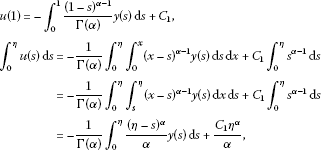yields

${C}_{1}={\int }_{0}^{1}\frac{{\left(1-s\right)}^{\alpha -1}}{\mathrm{\Gamma }\left(\alpha \right)\left(1-\frac{\lambda {\eta }^{\alpha }}{\alpha }\right)}y\left(s\right)\phantom{\rule{0.2em}{0ex}}\mathrm{d}s-\lambda {\int }_{0}^{\eta }\frac{{\left(\eta -s\right)}^{\alpha }}{\alpha \mathrm{\Gamma }\left(\alpha \right)\left(1-\frac{\lambda {\eta }^{\alpha }}{\alpha }\right)}y\left(s\right)\phantom{\rule{0.2em}{0ex}}\mathrm{d}s.$

Therefore, the unique solution of the problem (2) is

$\begin{array}{rcl}u\left(t\right)& =& -{\int }_{0}^{t}\frac{{\left(t-s\right)}^{\alpha -1}}{\mathrm{\Gamma }\left(\alpha \right)}y\left(s\right)\phantom{\rule{0.2em}{0ex}}\mathrm{d}s+\frac{1}{\left(1-\frac{\lambda {\eta }^{\alpha }}{\alpha }\right)}{\int }_{0}^{1}\frac{{\left(1-s\right)}^{\alpha -1}{t}^{\alpha -1}}{\mathrm{\Gamma }\left(\alpha \right)}y\left(s\right)\phantom{\rule{0.2em}{0ex}}\mathrm{d}s\\ -\frac{1}{\left(1-\frac{\lambda {\eta }^{\alpha }}{\alpha }\right)}{\int }_{0}^{\eta }\frac{\frac{\lambda }{\alpha }{\left(\eta -s\right)}^{\alpha }{t}^{\alpha -1}}{\mathrm{\Gamma }\left(\alpha \right)}y\left(s\right)\phantom{\rule{0.2em}{0ex}}\mathrm{d}s.\end{array}$

For $t\le \eta$, one has

$\begin{array}{rcl}u\left(t\right)& =& -{\int }_{0}^{t}\frac{{\left(t-s\right)}^{\alpha -1}}{\mathrm{\Gamma }\left(\alpha \right)}y\left(s\right)\phantom{\rule{0.2em}{0ex}}\mathrm{d}s+\frac{1}{\left(1-\frac{\lambda }{\alpha }{\eta }^{\alpha }\right)}\left[\left({\int }_{0}^{t}+{\int }_{t}^{\eta }+{\int }_{\eta }^{1}\right)\frac{{\left(1-s\right)}^{\alpha -1}{t}^{\alpha -1}}{\mathrm{\Gamma }\left(\alpha \right)}y\left(s\right)\phantom{\rule{0.2em}{0ex}}\mathrm{d}s\right]\\ -\frac{\lambda }{\left(1-\frac{\lambda }{\alpha }{\eta }^{\alpha }\right)}\left[\left({\int }_{0}^{t}+{\int }_{t}^{\eta }\right)\frac{\frac{1}{\alpha }{\left(\eta -s\right)}^{\alpha }{t}^{\alpha -1}}{\mathrm{\Gamma }\left(\alpha \right)}y\left(s\right)\phantom{\rule{0.2em}{0ex}}\mathrm{d}s\right]\\ =& {\int }_{0}^{t}\frac{{t}^{\alpha -1}{\left(1-s\right)}^{\alpha -1}-\frac{\lambda }{\alpha }{\left(\eta -s\right)}^{\alpha }{t}^{\alpha -1}-\left(1-\frac{\lambda }{\alpha }{\eta }^{\alpha }\right){\left(t-s\right)}^{\alpha -1}}{\left(1-\frac{\lambda }{\alpha }{\eta }^{\alpha }\right)\mathrm{\Gamma }\left(\alpha \right)}y\left(s\right)\phantom{\rule{0.2em}{0ex}}\mathrm{d}s\\ +{\int }_{t}^{\eta }\frac{{t}^{\alpha -1}{\left(1-s\right)}^{\alpha -1}-\frac{\lambda }{\alpha }{\left(\eta -s\right)}^{\alpha }{t}^{\alpha -1}}{\left(1-\frac{\lambda }{\alpha }{\eta }^{\alpha }\right)\mathrm{\Gamma }\left(\alpha \right)}y\left(s\right)\phantom{\rule{0.2em}{0ex}}\mathrm{d}s+{\int }_{\eta }^{1}\frac{{t}^{\alpha -1}{\left(1-s\right)}^{\alpha -1}}{\left(1-\frac{\lambda }{\alpha }{\eta }^{\alpha }\right)\mathrm{\Gamma }\left(\alpha \right)}y\left(s\right)\phantom{\rule{0.2em}{0ex}}\mathrm{d}s\\ =& {\int }_{0}^{1}G\left(t,s\right)y\left(s\right)\phantom{\rule{0.2em}{0ex}}\mathrm{d}s.\end{array}$

For $t\ge \eta$, one has

$\begin{array}{rcl}u\left(t\right)& =& -\left({\int }_{0}^{\eta }+{\int }_{\eta }^{t}\right)\frac{{\left(t-s\right)}^{\alpha -1}}{\mathrm{\Gamma }\left(\alpha \right)}y\left(s\right)\phantom{\rule{0.2em}{0ex}}\mathrm{d}s\\ +\frac{1}{\left(1-\frac{\lambda }{\alpha }{\eta }^{\alpha }\right)}\left[\left({\int }_{0}^{\eta }+{\int }_{\eta }^{t}+{\int }_{t}^{1}\right)\frac{{\left(1-s\right)}^{\alpha -1}{t}^{\alpha -1}}{\mathrm{\Gamma }\left(\alpha \right)}y\left(s\right)\phantom{\rule{0.2em}{0ex}}\mathrm{d}s\right]\\ -\frac{\lambda }{\left(1-\frac{\lambda }{\alpha }{\eta }^{\alpha }\right)}{\int }_{0}^{\eta }\frac{\frac{1}{\alpha }{\left(\eta -s\right)}^{\alpha }{t}^{\alpha -1}}{\mathrm{\Gamma }\left(\alpha \right)}y\left(s\right)\phantom{\rule{0.2em}{0ex}}\mathrm{d}s\\ =& {\int }_{0}^{\eta }\frac{{t}^{\alpha -1}{\left(1-s\right)}^{\alpha -1}-\frac{\lambda }{\alpha }{\left(\eta -s\right)}^{\alpha }{t}^{\alpha -1}-\left(1-\frac{\lambda }{\alpha }{\eta }^{\alpha }\right){\left(t-s\right)}^{\alpha -1}}{\left(1-\frac{\lambda }{\alpha }{\eta }^{\alpha }\right)\mathrm{\Gamma }\left(\alpha \right)}y\left(s\right)\phantom{\rule{0.2em}{0ex}}\mathrm{d}s\\ +{\int }_{\eta }^{t}\frac{{t}^{\alpha -1}{\left(1-s\right)}^{\alpha -1}-\left(1-\frac{\lambda }{\alpha }{\eta }^{\alpha }\right){\left(t-s\right)}^{\alpha -1}}{\left(1-\frac{\lambda }{\alpha }{\eta }^{\alpha }\right)\mathrm{\Gamma }\left(\alpha \right)}y\left(s\right)\phantom{\rule{0.2em}{0ex}}\mathrm{d}s+{\int }_{t}^{1}\frac{{t}^{\alpha -1}{\left(1-s\right)}^{\alpha -1}}{\left(1-\frac{\lambda }{\alpha }{\eta }^{\alpha }\right)\mathrm{\Gamma }\left(\alpha \right)}y\left(s\right)\phantom{\rule{0.2em}{0ex}}\mathrm{d}s\\ =& {\int }_{0}^{1}G\left(t,s\right)y\left(s\right)\phantom{\rule{0.2em}{0ex}}\mathrm{d}s.\end{array}$

The proof is complete. □

Lemma 2.4 The function$G\left(t,s\right)$defined by (3) satisfies

(a1) $G\left(t,s\right)\ge {m}_{1}e\left(t\right)s{\left(1-s\right)}^{\alpha -1}$, $\mathrm{\forall }t,s\in \left[0,1\right]$;

(a2) $G\left(t,s\right)\le {M}_{1}e\left(t\right){\left(1-s\right)}^{\alpha -1}$, $\mathrm{\forall }t,s\in \left[0,1\right]$;

(a3) $G\left(t,s\right)\le {M}_{1}s{\left(1-s\right)}^{\alpha -1}$, $\mathrm{\forall }t,s\in \left[0,1\right]$;

(a4) $p\left(s\right)>0$, and$p\left(s\right)$is not decreasing on$\left[0,1\right]$;

(a5) $G\left(t,s\right)>0$, $\mathrm{\forall }t,s\in \left(0,1\right)$,

where${m}_{1}=\frac{1-p\left(0\right)}{\mathrm{\Gamma }\left(\alpha \right)p\left(0\right)}$, ${M}_{1}=\frac{\alpha -1}{\mathrm{\Gamma }\left(\alpha \right)}+\frac{4\frac{\lambda }{\alpha }{\eta }^{\alpha -1}}{p\left(0\right)\mathrm{\Gamma }\left(\alpha \right)}$, $e\left(t\right)={t}^{\alpha -1}$.

Proof For $s\le t$, $s\le \eta$,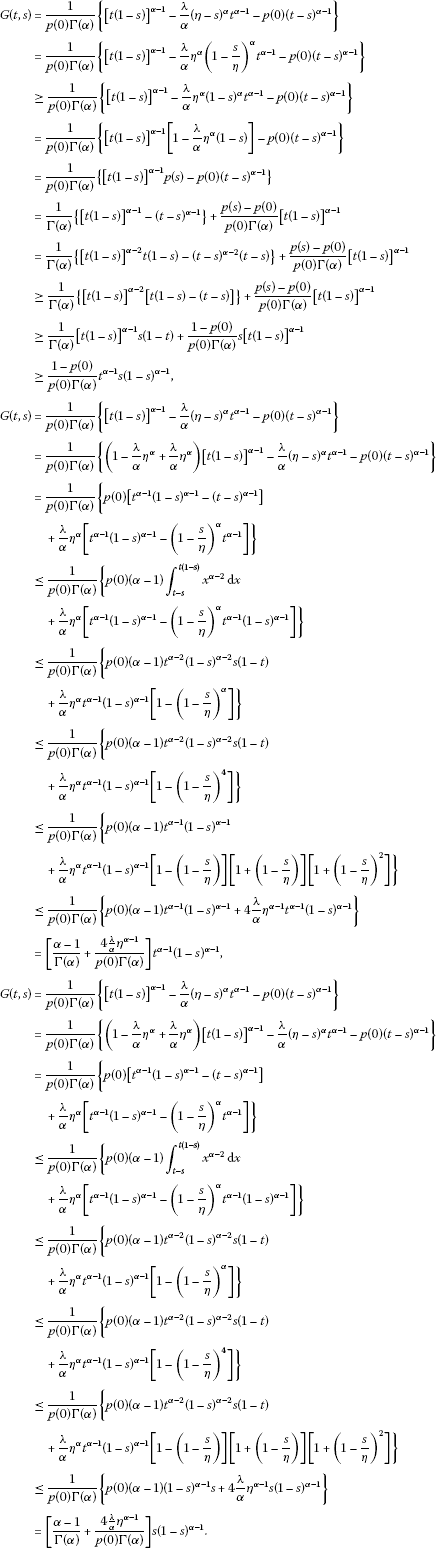For $\eta \le s\le t$,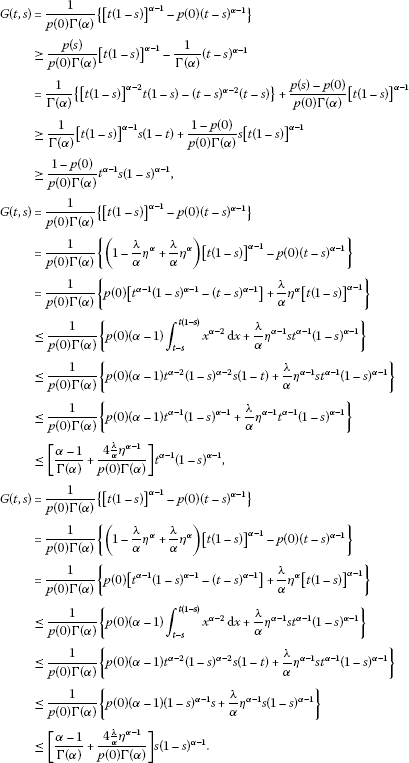For $t\le s\le \eta$,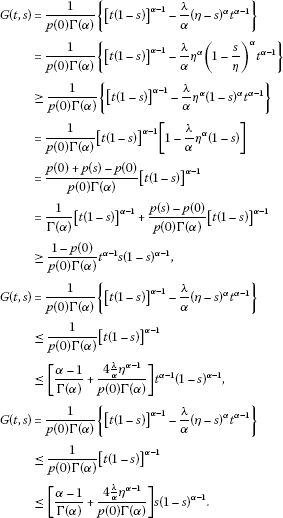For $\eta \le s$, $t\le s$,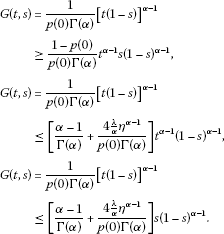From above, (a1), (a2), (a3), (a5) are complete. Clearly, (a4) is true. The proof is complete. □

(H1) $f\in C\left[\left(0,1\right)×\left[0,+\mathrm{\infty }\right),\left(-\mathrm{\infty },+\mathrm{\infty }\right)\right]$ and there exist $a,b\in C\left[J,{R}^{+}\right]$, $h\in C\left[{R}^{+},{R}^{+}\right]$, $g\left(t,u\right)\in \left[J×{R}^{+},{R}^{+}\right]$ such that

$g\left(t,u\right)-b\left(t\right)\le f\left(t,u\right)\le a\left(t\right)h\left(u\right),\phantom{\rule{1em}{0ex}}\mathrm{\forall }t\in J,u\in {R}^{+};$

(H2) There exists $\left[a,b\right]\subset I$ such that ${lim}_{u\to +\mathrm{\infty }}\frac{g\left(t,u\right)}{u}=+\mathrm{\infty }$ uniformly for $t\in \left[a,b\right]$;

(H3) There exists $r>0$ such that

$\begin{array}{c}{\int }_{0}^{1}{\left(1-s\right)}^{\alpha -1}b\left(s\right)\phantom{\rule{0.2em}{0ex}}\mathrm{d}s<\frac{{m}_{1}}{{M}_{1}^{2}}r,\hfill \\ {M}_{1}{\int }_{0}^{1}{\left(1-s\right)}^{\alpha -1}\left(a\left(s\right)+b\left(s\right)\right)\phantom{\rule{0.2em}{0ex}}\mathrm{d}s<\frac{r}{{max}_{0\le u\le r}\left\{h\left(u\right),1\right\}}.\hfill \end{array}$

Let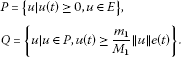(4)

Obviously, Q is a cone in a Banach space E and $\left(E,Q\right)$ is an ordering Banach space.

Let

${x}_{0}\left(t\right)={\int }_{0}^{1}G\left(t,s\right)b\left(s\right)\phantom{\rule{0.2em}{0ex}}\mathrm{d}s,\phantom{\rule{1em}{0ex}}t\in I,$
(5)

where $b\left(t\right)$ is defined as that in (H1). It follows from Lemma 2.4 and (H3) that

$0\le {x}_{0}\left(t\right)\le {M}_{1}e\left(t\right){\int }_{0}^{1}{\left(1-s\right)}^{\alpha -1}b\left(s\right)\phantom{\rule{0.2em}{0ex}}\mathrm{d}s<+\mathrm{\infty },\phantom{\rule{1em}{0ex}}t\in I.$
(6)

So, ${x}_{0}\in P$ and it satisfies

$\left\{\begin{array}{c}{D}_{0+}^{\alpha }{x}_{0}\left(t\right)+{x}_{0}\left(t\right)=0,\phantom{\rule{1em}{0ex}}0
(7)

For any $u\in Q\mathrm{\setminus }\left\{\theta \right\}$, $u\left(t\right)\ge \frac{{m}_{1}}{{M}_{1}}\parallel u\parallel e\left(t\right)$, $t\in I$. Consequently, by (6) and Lemma 2.4, we have

$\begin{array}{rcl}{x}_{0}\left(t\right)& \le & {M}_{1}e\left(t\right){\int }_{0}^{1}{\left(1-s\right)}^{\alpha -1}b\left(s\right)\phantom{\rule{0.2em}{0ex}}\mathrm{d}s\\ \le & {M}_{1}\parallel u\parallel \frac{{m}_{1}}{{M}_{1}}e\left(t\right)\cdot \frac{1}{\parallel u\parallel \frac{{m}_{1}}{{M}_{1}}}{\int }_{0}^{1}{\left(1-s\right)}^{\alpha -1}b\left(s\right)\phantom{\rule{0.2em}{0ex}}\mathrm{d}s\\ \le & \frac{u\left(t\right)}{\parallel u\parallel }\frac{{M}_{1}^{2}}{{m}_{1}}{\int }_{0}^{1}{\left(1-s\right)}^{\alpha -1}b\left(s\right)\phantom{\rule{0.2em}{0ex}}\mathrm{d}s.\end{array}$
(8)

For any $k\in E$, denote

${\left[k\left(t\right)\right]}^{+}=\left\{\begin{array}{cc}k\left(t\right),\hfill & k\left(t\right)\ge 0,\hfill \\ 0,\hfill & k\left(t\right)<0.\hfill \end{array}$

We define an operator A as follows:

$\left(Au\right)\left(t\right)={\int }_{0}^{1}G\left(t,s\right)\left(f\left(s,{\left[u\left(s\right)-{x}_{0}\left(s\right)\right]}^{+}\right)+b\left(s\right)\right)\phantom{\rule{0.2em}{0ex}}\mathrm{d}s,\phantom{\rule{1em}{0ex}}\mathrm{\forall }u\in P.$
(9)

Lemma 2.5 Suppose that (${\mathrm{H}}_{1}$)-(${\mathrm{H}}_{3}$) hold. Then$A:Q\to Q$is completely continuous.

Proof For any $u\in P$, it is clear that ${\left[u\left(s\right)-{x}_{0}\left(s\right)\right]}^{+}\le u\left(s\right)\le \parallel u\parallel$. By (H1), we get

$\begin{array}{rcl}f\left(s,{\left[u\left(s\right)-{x}_{0}\left(s\right)\right]}^{+}\right)+b\left(s\right)& \le & a\left(s\right)h\left({\left[u\left(s\right)-{x}_{0}\left(s\right)\right]}^{+}\right)+b\left(s\right)\\ \le & \underset{0\le r\le \parallel u\parallel }{max}\left\{h\left(r\right),1\right\}\left(a\left(s\right)+b\left(s\right)\right),\phantom{\rule{1em}{0ex}}\mathrm{\forall }s\in J.\end{array}$
(10)

By (10) and Lemma 2.4, we have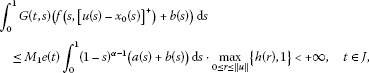(11)

which together with (H3) means that operator A defined by (9) is well defined.

Now, we show that $A:Q\to Q$.

For any $u\in Q$, by (H1) we have by (9) and Lemma 2.4 that

$\left(Au\right)\left(t\right)\le {M}_{1}{\int }_{0}^{1}s{\left(1-s\right)}^{\alpha -1}\left[f\left(s,{\left[u\left(s\right)-{x}_{0}\left(s\right)\right]}^{+}\right)+b\left(s\right)\right]\phantom{\rule{0.2em}{0ex}}\mathrm{d}s,\phantom{\rule{1em}{0ex}}\mathrm{\forall }t\in I,$

which means that

$\parallel Au\parallel \le {M}_{1}{\int }_{0}^{1}s{\left(1-s\right)}^{\alpha -1}\left[f\left(s,{\left[u\left(s\right)-{x}_{0}\left(s\right)\right]}^{+}\right)+b\left(s\right)\right]\phantom{\rule{0.2em}{0ex}}\mathrm{d}s.$
(12)

It follows from (12) and Lemma 2.4 that

$\begin{array}{rcl}\left(Au\right)\left(t\right)& =& {\int }_{0}^{1}G\left(t,s\right)\left(f\left(s,{\left[u\left(s\right)-{x}_{0}\left(s\right)\right]}^{+}\right)+b\left(s\right)\right)\phantom{\rule{0.2em}{0ex}}\mathrm{d}s\\ \ge & {m}_{1}e\left(t\right){\int }_{0}^{1}s{\left(1-s\right)}^{\alpha -1}\left[f\left(s,{\left[u\left(s\right)-{x}_{0}\left(s\right)\right]}^{+}\right)+b\left(s\right)\right]\phantom{\rule{0.2em}{0ex}}\mathrm{d}s\\ =& \frac{{m}_{1}}{{M}_{1}}e\left(t\right){\int }_{0}^{1}{M}_{1}s{\left(1-s\right)}^{\alpha -1}\left[f\left(s,{\left[u\left(s\right)-{x}_{0}\left(s\right)\right]}^{+}\right)+b\left(s\right)\right]\phantom{\rule{0.2em}{0ex}}\mathrm{d}s\\ \ge & \frac{{m}_{1}}{{M}_{1}}e\left(t\right)\parallel Au\parallel .\end{array}$

Thus, A maps Q into Q.

Finally, we prove that A maps Q into Q is completely continuous.

Let $D\subset Q$ be any bounded set. Then there exists a constant ${L}_{1}>0$ such that $\parallel u\parallel \le {L}_{1}$ for any $u\in D$. Notice that ${\left[u\left(s\right)-{x}_{0}\left(s\right)\right]}^{+}\le u\left(s\right)\le {L}_{1}$, for any $u\in D$, $s\in I$, by (H3) and (11), we have

$|\left(Au\right)\left(t\right)|\le {M}_{1}{\int }_{0}^{1}{\left(1-s\right)}^{\alpha -1}\left(a\left(s\right)+b\left(s\right)\right)\phantom{\rule{0.2em}{0ex}}\mathrm{d}s\cdot \underset{0\le r\le {L}_{1}}{max}\left\{h\left(r\right),1\right\}<+\mathrm{\infty }.$

Therefore, $A\left(D\right)$ is uniformly bounded.

On the other hand, since $G\left(t,s\right)$ is continuous on $\left[0,1\right]×\left[0,1\right]$, it is uniformly continuous on $\left[0,1\right]×\left[0,1\right]$ as well. Thus, for fixed $s\in I$ and for any $\epsilon >0$, there exists a constant $\delta >0$ such that for any ${t}_{1},{t}_{2}\in \left[0,1\right]$ and $|{t}_{1}-{t}_{2}|<\delta$,

$|G\left({t}_{1},s\right)-G\left({t}_{2},s\right)|<\frac{\epsilon }{{max}_{0\le r\le {L}_{1}}\left\{h\left(r\right),1\right\}{\int }_{0}^{1}\left(a\left(s\right)+b\left(s\right)\right)\phantom{\rule{0.2em}{0ex}}\mathrm{d}s}.$
(13)

Therefore, for any $x\in D$, we get by (10) and (13)

$\begin{array}{c}|\left(Au\right)\left({t}_{1}\right)-\left(Au\right)\left({t}_{2}\right)|\hfill \\ \phantom{\rule{1em}{0ex}}\le {\int }_{0}^{1}|G\left({t}_{1},s\right)-G\left({t}_{2},s\right)|\left(f\left(s,{\left[u\left(s\right)-{x}_{0}\left(s\right)\right]}^{+}\right)+b\left(s\right)\right)\phantom{\rule{0.2em}{0ex}}\mathrm{d}s\hfill \\ \phantom{\rule{1em}{0ex}}\le \frac{\epsilon }{{max}_{0\le r\le {L}_{1}}\left\{h\left(r\right),1\right\}{\int }_{0}^{1}\left(a\left(s\right)+b\left(s\right)\right)\phantom{\rule{0.2em}{0ex}}\mathrm{d}s}{\int }_{0}^{1}\left(a\left(s\right)+b\left(s\right)\right)\phantom{\rule{0.2em}{0ex}}\mathrm{d}s\cdot \underset{0\le r\le {L}_{1}}{max}\left\{h\left(r\right),1\right\}=\epsilon ,\hfill \end{array}$

which implies that the operator A is equicontinuous. Thus, the Ascoli-Arzela theorem guarantees that $A\left(D\right)$ is a relatively compact set.

Let ${u}_{n},{u}_{0}\in Q$, ${u}_{n}\to {u}_{0}$ ($n\to \mathrm{\infty }$). Then $\left\{{u}_{n}\right\}$ is bounded. Let ${L}_{2}=sup\left\{\parallel {u}_{n}\parallel ,n=0,1,2,\dots \right\}$, by (10), we get

$f\left(s,{\left[{u}_{n}\left(s\right)-{x}_{0}\left(s\right)\right]}^{+}\right)+b\left(s\right)\le \left(\underset{0\le r\le {L}_{2}}{max}\left\{h\left(r\right),1\right\}\right)\left(a\left(s\right)+b\left(s\right)\right),\phantom{\rule{1em}{0ex}}n=0,1,2,\dots .$
(14)

By (9), we have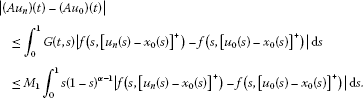(15)

It follows from (14), (15), (H1), (H3), and the Lebesgue dominated convergence theorem that A is continuous. Thus, we have proved the continuity of the operator A. This completes the complete continuity of A. □

To prove the main result, we need the following well-known fixed point theorem.

Lemma 2.6 (Fixed point theorem of cone expansion and compression of norm type )

Let${\mathrm{\Omega }}_{1}$and${\mathrm{\Omega }}_{2}$be two bounded open sets in a Banach space E such that$\theta \in {\mathrm{\Omega }}_{1}$and${\overline{\mathrm{\Omega }}}_{1}\subset {\mathrm{\Omega }}_{2},A:P\cap \left({\overline{\mathrm{\Omega }}}_{2}\mathrm{\setminus }{\mathrm{\Omega }}_{1}\right)\to P$be a completely continuous operator, where θ denotes the zero element of E and P a cone of E. Suppose that one of the two conditions holds:

1. (i)

$\parallel Au\parallel \le \parallel u\parallel$, $\mathrm{\forall }u\in P\cap \partial {\mathrm{\Omega }}_{1}$; $\parallel Au\parallel \ge \parallel u\parallel$, $\mathrm{\forall }u\in P\cap \partial {\mathrm{\Omega }}_{2}$;

2. (ii)

$\parallel Au\parallel \ge \parallel u\parallel$, $\mathrm{\forall }u\in P\cap \partial {\mathrm{\Omega }}_{1}$; $\parallel Au\parallel \le \parallel u\parallel$, $\mathrm{\forall }u\in P\cap \partial {\mathrm{\Omega }}_{2}$.

Then A has a fixed point in$P\cap \left({\overline{\mathrm{\Omega }}}_{2}\mathrm{\setminus }{\mathrm{\Omega }}_{1}\right)$.

## 3 Main result

Theorem 3.1 Assume that conditions (${\mathrm{H}}_{1}$)-(${\mathrm{H}}_{3}$) are satisfied. Then the singular semipositone BVP (1) has at least one positive solution$\omega \left(t\right)$. Furthermore, there exist two constants$M>m>0$such that

$me\left(t\right)\le \omega \left(t\right)\le Me\left(t\right),\phantom{\rule{1em}{0ex}}t\in I.$
(16)

Proof Firstly, we show that the operator A has a fixed point in Q. Let

${\mathrm{\Omega }}_{r}=\left\{u\in E|\parallel u\parallel

where r is the same as that defined in (H3). For any $u\in \partial {\mathrm{\Omega }}_{r}\cap Q$, by (10) and (12), we have that

$\begin{array}{rcl}\left(Au\right)\left(t\right)& =& {\int }_{0}^{1}G\left(t,s\right)\left(f\left(s,{\left[u\left(s\right)-{x}_{0}\left(s\right)\right]}^{+}\right)+b\left(s\right)\right)\phantom{\rule{0.2em}{0ex}}\mathrm{d}s\\ \le & {M}_{1}{\int }_{0}^{1}s{\left(1-s\right)}^{\alpha -1}\left(a\left(s\right)+b\left(s\right)\right)\phantom{\rule{0.2em}{0ex}}\mathrm{d}s\cdot \underset{0\le u\le r}{max}\left\{h\left(u\right),1\right\}<+\mathrm{\infty },\phantom{\rule{1em}{0ex}}t\in J.\end{array}$

Therefore,

$\parallel Au\parallel \le {M}_{1}{\int }_{0}^{1}s{\left(1-s\right)}^{\alpha -1}\left(a\left(s\right)+b\left(s\right)\right)\phantom{\rule{0.2em}{0ex}}\mathrm{d}s\cdot \underset{0\le u\le r}{max}\left\{h\left(u\right),1\right\}

which together with (H3) implies that

$\parallel Au\parallel \le r=\parallel u\parallel ,\phantom{\rule{1em}{0ex}}\mathrm{\forall }u\in \partial {\mathrm{\Omega }}_{r}\cap Q.$
(17)

For $\left[a,b\right]$ in (H2), it is clear that

$e\left(t\right)\ge {a}^{\alpha -1},\phantom{\rule{1em}{0ex}}t\in \left[a,b\right].$
(18)

By (H3), we know that there exists a natural number ${m}_{0}$ big enough such that

${\int }_{0}^{1}{\left(1-s\right)}^{\alpha -1}b\left(s\right)\phantom{\rule{0.2em}{0ex}}\mathrm{d}s<\frac{{m}_{0}}{{m}_{0}+1}\frac{{m}_{1}}{{M}_{1}^{2}}r.$
(19)

Choose

$M>\frac{{M}_{1}\left({m}_{0}+1\right)}{{m}_{1}{a}^{\alpha -1}{min}_{t\in \left[a,b\right]}{\int }_{0}^{1}G\left(t,s\right)\phantom{\rule{0.2em}{0ex}}\mathrm{d}s}.$
(20)

By (H2), we know there exists ${R}_{1}>r$ such that

$g\left(t,u\right)\ge Mu,\phantom{\rule{1em}{0ex}}\mathrm{\forall }t\in \left[a,b\right],u\ge {R}_{1}.$
(21)

Take

$R>max\left\{r,1,\frac{{R}_{1}\left({m}_{0}+1\right){M}_{1}}{{a}^{\alpha -1}{m}_{1}}\right\}.$
(22)

In the following, we are in a position to show that

$\parallel Au\parallel \ge \parallel u\parallel ,\phantom{\rule{1em}{0ex}}\mathrm{\forall }u\in \partial {\mathrm{\Omega }}_{R}\cap Q.$
(23)

For any $u\in \partial {\mathrm{\Omega }}_{R}\cap Q$, by (8) we get

${x}_{0}\left(t\right)\le \frac{u\left(t\right)}{\parallel u\parallel }\frac{{M}_{1}^{2}}{{m}_{1}}{\int }_{0}^{1}{\left(1-s\right)}^{\alpha -1}b\left(s\right)\phantom{\rule{0.2em}{0ex}}\mathrm{d}s,$

which together with (18), (19), (22), and (H3) implies that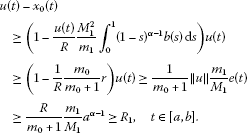(24)

For $u\in \partial {\mathrm{\Omega }}_{R}\cap Q$, $t\in \left[a,b\right]$, it follows from (H1), (20), (21), (22), and (24) that

$\begin{array}{rcl}\left(Au\right)\left(t\right)& =& {\int }_{0}^{1}G\left(t,s\right)\left(f\left(s,{\left[u\left(s\right)-{x}_{0}\left(s\right)\right]}^{+}\right)+b\left(s\right)\right)\phantom{\rule{0.2em}{0ex}}\mathrm{d}s\\ \ge & {\int }_{0}^{1}G\left(t,s\right)g\left(s,{\left[u\left(s\right)-{x}_{0}\left(s\right)\right]}^{+}\right)\phantom{\rule{0.2em}{0ex}}\mathrm{d}s\\ \ge & {\int }_{0}^{1}G\left(t,s\right)M\left(u\left(s\right)-{x}_{0}\left(s\right)\right)\phantom{\rule{0.2em}{0ex}}\mathrm{d}s\\ \ge & M\frac{R}{{m}_{0}+1}\frac{{m}_{1}}{{M}_{1}}{a}^{\alpha -1}\underset{t\in \left[a,b\right]}{min}{\int }_{0}^{1}G\left(t,s\right)\phantom{\rule{0.2em}{0ex}}\mathrm{d}s>R,\phantom{\rule{1em}{0ex}}t\in \left[a,b\right].\end{array}$
(25)

By (25), we know that (23) holds. So, (17), (23), and Lemma 2.6 guarantee that A has at least one fixed point ${z}_{0}\left(t\right)$ in $\left({\overline{\mathrm{\Omega }}}_{R}\mathrm{\setminus }{\mathrm{\Omega }}_{r}\right)\cap Q$ and $r\le \parallel {z}_{0}\parallel \le R$. Furthermore,

${z}_{0}\left(t\right)={\int }_{0}^{1}G\left(t,s\right)\left(f\left(s,{\left[{z}_{0}\left(s\right)-{x}_{0}\left(s\right)\right]}^{+}\right)+b\left(s\right)\right)\phantom{\rule{0.2em}{0ex}}\mathrm{d}s,\phantom{\rule{1em}{0ex}}t\in I.$
(26)

By simple computation, we have that

$\left\{\begin{array}{c}{D}_{0+}^{\alpha }{z}_{0}\left(t\right)+f\left(t,{\left[{z}_{0}\left(t\right)-{x}_{0}\left(t\right)\right]}^{+}\right)+b\left(t\right)=0,\phantom{\rule{1em}{0ex}}0
(27)

Secondly, we show BVP (1) has a positive solution. It follows from (8) and the fact $\parallel {z}_{0}\parallel \ge r$ that

${x}_{0}\left(t\right)\le \frac{{z}_{0}\left(t\right)}{\parallel {z}_{0}\parallel }\frac{{M}_{1}^{2}}{{m}_{1}}{\int }_{0}^{1}{\left(1-s\right)}^{\alpha -1}b\left(s\right)\phantom{\rule{0.2em}{0ex}}\mathrm{d}s\le \frac{{z}_{0}\left(t\right)}{r}\frac{{M}_{1}^{2}}{{m}_{1}}{\int }_{0}^{1}{\left(1-s\right)}^{\alpha -1}b\left(s\right)\phantom{\rule{0.2em}{0ex}}\mathrm{d}s,$

which combined with (19) implies that

${z}_{0}\left(t\right)-{x}_{0}\left(t\right)\ge \left(1-\frac{1}{r}\frac{{M}_{1}^{2}}{{m}_{1}}{\int }_{0}^{1}{\left(1-s\right)}^{\alpha -1}b\left(s\right)\phantom{\rule{0.2em}{0ex}}\mathrm{d}s\right){z}_{0}\left(t\right)\ge \frac{1}{{m}_{0}+1}{z}_{0}\left(t\right)\ge 0.$
(28)

By (27) and (28), we have

$\left\{\begin{array}{c}{D}_{0+}^{\alpha }{z}_{0}\left(t\right)+f\left(t,\left[{z}_{0}\left(t\right)-{x}_{0}\left(t\right)\right]\right)+b\left(t\right)=0,\phantom{\rule{1em}{0ex}}0
(29)

Let $\omega \left(t\right)={z}_{0}\left(t\right)-{x}_{0}\left(t\right)$, $t\in I$. It follows from (28) and ${z}_{0}\in Q$ that

$\omega \left(t\right)\ge \frac{1}{{m}_{0}+1}{z}_{0}\left(t\right)\ge \frac{{m}_{1}r}{\left({m}_{0}+1\right){M}_{1}}e\left(t\right)>0,\phantom{\rule{1em}{0ex}}t\in J.$
(30)

By (7), (29), and (30), we obtain

$\left\{\begin{array}{c}{D}_{0+}^{\alpha }\omega \left(t\right)+f\left(t,\omega \left(t\right)\right)=0,\phantom{\rule{1em}{0ex}}0

Thus, we have proved that $\omega \left(t\right)$ is a positive solution for BVP (1).

Finally, we show that (16) holds. From (26) and Lemma 2.4, we know that

${z}_{0}\left(t\right)\le {M}_{1}e\left(t\right){\int }_{0}^{1}{\left(1-s\right)}^{\alpha -1}\left(f\left(s,{\left[{z}_{0}\left(s\right)-{x}_{0}\left(s\right)\right]}^{+}\right)+b\left(s\right)\right)\phantom{\rule{0.2em}{0ex}}\mathrm{d}s,\phantom{\rule{1em}{0ex}}t\in I.$
(31)

Since $\omega \left(t\right)\le {z}_{0}\left(t\right)$, (30), and (31) mean that (16) holds for $m=\frac{{m}_{1}r}{\left({m}_{0}+1\right){M}_{1}}$ and $M={M}_{1}{\int }_{0}^{1}{\left(1-s\right)}^{\alpha -1}\left(f\left(s,{\left[{z}_{0}\left(s\right)-{x}_{0}\left(s\right)\right]}^{+}\right)+b\left(s\right)\right)\phantom{\rule{0.2em}{0ex}}\mathrm{d}s$ holds. This completes the proof of Theorem 3.1. □

## 4 Example

Consider the following singular semipositone fractional differential equations:

$\left\{\begin{array}{c}{D}_{0+}^{\frac{7}{2}}u\left(t\right)+f\left(t,u\left(t\right)\right)=0,\phantom{\rule{1em}{0ex}}0
(32)

where $f\left(t,u\right)=\frac{{t}^{2}}{8\sqrt{1-t}}{u}^{\frac{5}{2}}+\frac{{t}^{\frac{1}{4}}}{20\left(1-t\right)}\left(u-{u}^{\frac{1}{2}}-\frac{3}{4}\right)$. It is clear (32) has the form of (1), where $\alpha =\frac{7}{2}$, $\lambda =16\sqrt{2}$, $\eta =\frac{1}{2}$. By simple computation, we know that $0<\frac{\lambda {\eta }^{\alpha }}{\alpha }\approx 0.5714<1$, $p\left(0\right)\approx 0.4286$. Let

$\begin{array}{c}a\left(t\right)=\frac{{t}^{2}}{8\sqrt{1-t}}+\frac{{t}^{\frac{1}{4}}}{20\left(1-t\right)},\phantom{\rule{2em}{0ex}}b\left(t\right)=\frac{{t}^{\frac{1}{4}}}{20\left(1-t\right)},\hfill \\ g\left(t,u\right)=\frac{{t}^{2}}{8\sqrt{1-t}}{u}^{\frac{5}{2}},\phantom{\rule{2em}{0ex}}h\left(u\right)={u}^{\frac{5}{2}}+u-{u}^{\frac{1}{2}}+\frac{1}{4}.\hfill \end{array}$

Notice that

$-1\le u-{u}^{\frac{1}{2}}-\frac{3}{4}\le u-{u}^{\frac{1}{2}}+\frac{1}{4},$

we have

$-\frac{{t}^{\frac{1}{4}}}{20\left(1-t\right)}\le \frac{{t}^{\frac{1}{4}}}{20\left(1-t\right)}\left(u-{u}^{\frac{1}{2}}-\frac{3}{4}\right)\le \frac{{t}^{\frac{1}{4}}}{20\left(1-t\right)}\left(u-{u}^{\frac{1}{2}}+\frac{1}{4}\right).$
(33)

It follows from the left side of (33) that

$-b\left(t\right)\le f\left(t,u\right)-g\left(t,u\right).$
(34)

Considering $u-{u}^{\frac{1}{2}}+\frac{1}{4}\ge 0$, we get

$f\left(t,u\right)\le \frac{{t}^{2}}{8\sqrt{1-t}}{u}^{\frac{5}{2}}+\frac{{t}^{\frac{1}{4}}}{20\left(1-t\right)}\left(u-{u}^{\frac{1}{2}}+\frac{1}{4}\right)\le a\left(t\right)h\left(u\right).$
(35)

By (34) and (35) we know (H1) holds. Obviously, (H2) holds for $\left[a,b\right]=\left[\frac{1}{4},\frac{3}{4}\right]$.

Now, we check (H3). By simple computation, we have ${m}_{1}=0.4012$, ${M}_{1}=3.9619$, $\frac{{m}_{1}}{{M}_{1}^{2}}=0.0256$, ${\int }_{0}^{1}{\left(1-s\right)}^{\alpha -1}b\left(s\right)\phantom{\rule{0.2em}{0ex}}\mathrm{d}s\approx 0.0136$, ${M}_{1}{\int }_{0}^{1}{\left(1-s\right)}^{\alpha -1}\left(a\left(s\right)+b\left(s\right)\right)\phantom{\rule{0.2em}{0ex}}\mathrm{d}s\approx 0.1245$. Take $r=1$, then ${max}_{0\le u\le r}\left\{h\left(u\right),1\right\}\approx 1.2500$, $\frac{r}{{max}_{0\le u\le r}\left\{h\left(u\right),1\right\}}\approx 0.8000$. Thus, (H3) is valid. It follows from Theorem 3.1 that BVP (32) has at least one positive solution.

## References

1. Anuradha V, Hai DD, Shivaji R: Existence results for superlinear semipositone BVP’s. Proc. Am. Math. Soc. 1996, 124: 747-763. doi:10.1090/S0002-9939-96-03256-X

2. Agarwal RP, O’Regan D: A note on existence of nonnegative solutions to singular semi-positone problems. Nonlinear Anal. 1999, 36: 615-622. doi:10.1016/S0362-546X(98)00181-3 10.1016/S0362-546X(98)00181-3

3. Xu X: Positive solutions for singular semi-positone boundary value problems. J. Math. Anal. Appl. 2002, 273: 480-491. doi:10.1016/S0022-247X(02)00259-7 10.1016/S0022-247X(02)00259-7

4. Zhao Z: Existence of positive solutions for 2 n th-order singular semipositone differential equations with Sturm-Liouville boundary conditions. Nonlinear Anal. 2010, 72: 1348-1357. doi:10.1016/j.na.2009.08.013 10.1016/j.na.2009.08.013

5. Ma R: Positive solutions for semipositone $\left(k,n-k\right)$ conjugate boundary value problems. J. Math. Anal. Appl. 2000, 252: 220-229. doi:10.1006/jmaa.2000.6987 10.1006/jmaa.2000.6987

6. Ma R, Ma Q: Positive solutions for semipositone m -point boundary-value problems. Acta Math. Sin. 2004, 20(2):273-282. doi:10.1007/s10114-003-0251-9 10.1007/s10114-003-0251-9

7. Su H, Liu L, Wu Y: Positive solutions for a nonlinear second-order semipositone boundary value system. Nonlinear Anal. 2009, 71: 3240-3248. doi:10.1016/j.na.2009.01.201 10.1016/j.na.2009.01.201

8. Liu Y: Twin solutions to singular semipositone problems. J. Math. Anal. Appl. 2003, 286: 248-260. doi:10.1016/S0022-247X(03)00478-5 10.1016/S0022-247X(03)00478-5

9. Zhang X, Liu L: Positive solutions of superlinear semipositone singular Dirichlet boundary value problems. J. Math. Anal. Appl. 2006, 316: 535-537. doi:10.1016/j.jmaa.2005.04.081

10. Zhang X, Liu L: Existence of positive solutions for a singular semipositone differential system. Math. Comput. Model. 2008, 47: 115-126. doi:10.1016/j.mcm.2007.02.008 10.1016/j.mcm.2007.02.008

11. Zhang X, Liu L, Wu Y: On existence of positive solutions of a two-point boundary value problem for a nonlinear singular semipositone system. Appl. Math. Comput. 2007, 192: 223-232. doi:10.1016/j.amc.2007.03.002 10.1016/j.amc.2007.03.002

12. Gallardo JM: Second order differential operators with integral boundary conditions and generation of semigroups. Rocky Mt. J. Math. 2000, 30: 1265-1292. doi:10.1216/rmjm/1021477351 10.1216/rmjm/1021477351

13. Karakostas GL, Tsamatos PC: Multiple positive solutions of some Fredholm integral equations arisen from nonlocal boundary-value problems. Electron. J. Differ. Equ. 2002., 2002: Article ID 30

14. Lomtatidze A, Malaguti L: On a nonlocal boundary-value problems for second order nonlinear singular differential equations. Georgian Math. J. 2000, 7: 133-154. doi:10.1515/GMJ.2000.133

15. Samko SG, Kilbas AA, Marichev OI: Fractional Integral and Derivative: Theory and Applications. Gordon & Breach, Switzerland; 1993.

16. Podlubny I Mathematics in Science and Engineering 198. In Fractional Differential Equations. Academic Press, New York; 1999.

17. Kilbas AA, Srivastava HM, Trujillo JJ North-Holland Mathematics Studies 204. In Theory and Applications of Fractional Differential Equations. Elsevier, Amsterdam; 2006.

18. Webb JRL: Nonlocal conjugate type boundary value problems of higher order. Nonlinear Anal. 2009, 71: 1933-1940. doi:10.1016/j.na.2009.01.033 10.1016/j.na.2009.01.033

19. Hao X, Liu L, Wu Y, Sun Q: Positive solutions for nonlinear n th-order singular eigenvalue problem with nonlocal conditions. Nonlinear Anal. 2010, 73: 1653-1662. doi:10.1016/j.na.2010.04.074 10.1016/j.na.2010.04.074

20. Wang Y, Liu L, Wu Y: Positive solutions for a nonlocal fractional differential equation. Nonlinear Anal. 2011, 74: 3599-3605. doi:10.1016/j.na.2011.02.043 10.1016/j.na.2011.02.043

21. Cabada A, Wang G: Positive solutions of nonlinear fractional differential equations with integral boundary value conditions. J. Math. Anal. Appl. 2012, 389: 403-411. doi:10.1016/j.jmaa.2011.11.065 10.1016/j.jmaa.2011.11.065

22. Guo D, Lakshmikantham V: Nonlinear Problems in Abstract Cones. Academic Press, San Diego; 1988.

## Acknowledgements

The author thanks the referee for his/her careful reading of the manuscript and useful suggestions. The project is supported financially by the Foundation for Outstanding Middle-Aged and Young Scientists of Shandong Province (Grant No. BS2010SF004), a Project of Shandong Province Higher Educational Science and Technology Program (Grant No. J10LA53, No. J11LA02), the China Postdoctoral Science Foundation (Grant No. 20110491154) and the National Natural Science Foundation of China (Grant No. 10971179).

## Author information

Authors

### Corresponding author

Correspondence to Xingqiu Zhang.

### Competing interests

The author declares that he has no competing interests.

## Rights and permissions

Reprints and Permissions

Zhang, X. Positive solution for a class of singular semipositone fractional differential equations with integral boundary conditions. Bound Value Probl 2012, 123 (2012). https://doi.org/10.1186/1687-2770-2012-123

• Accepted:

• Published:

• DOI: https://doi.org/10.1186/1687-2770-2012-123

### Keywords

• fractional differential equations
• integral boundary value problem
• positive solution
• semipositone
• cone